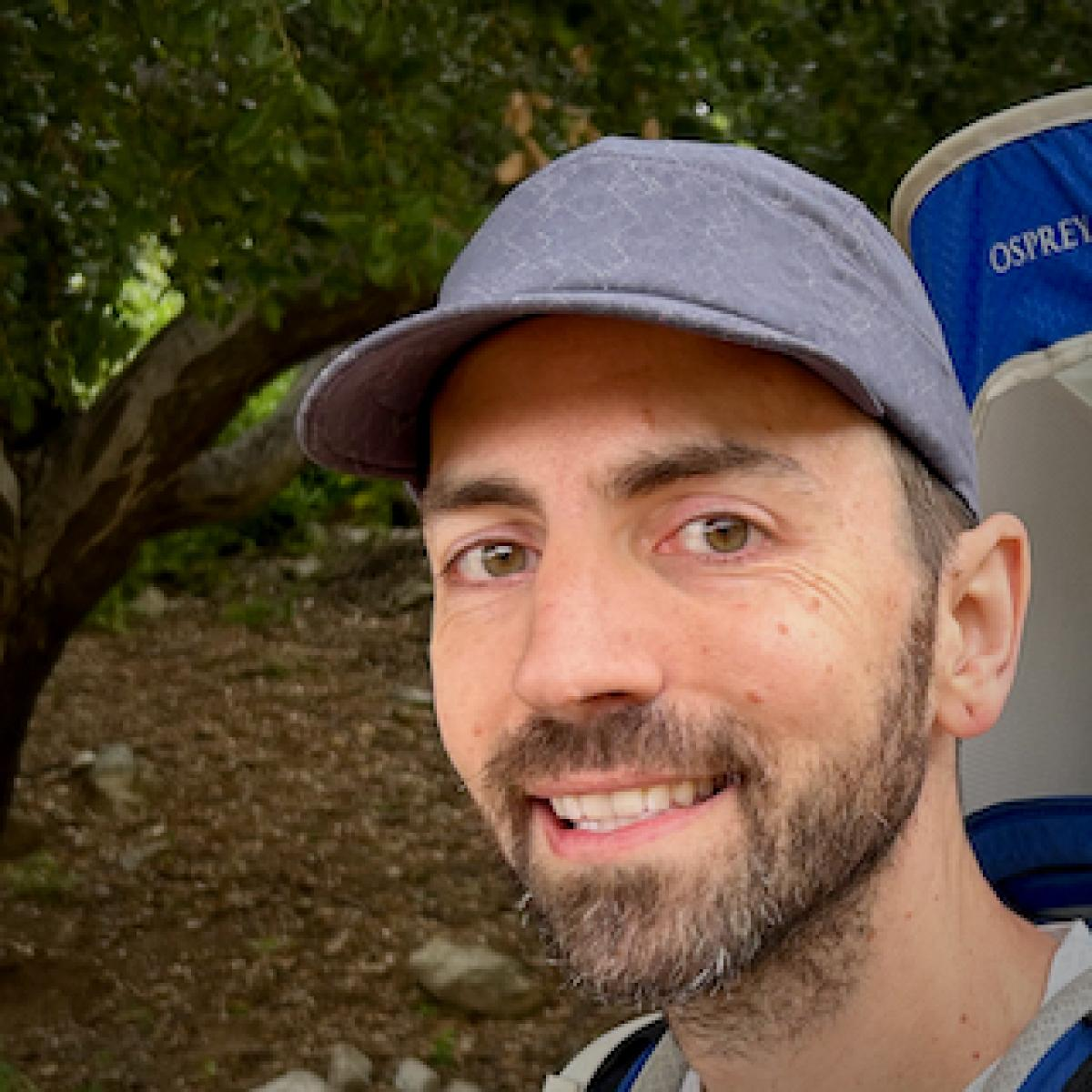# Steven Flammia

Research Scientist, AWS Center for Quantum Computing

Steven Flammia is a Principal Research Scientist at the AWS Center for Quantum Computing. He is a quantum information theorist interested in quantum error correcting codes, quantum fault tolerance, and learning quantum systems, particularly learning quantum noise. His research goals are to understand both the fundamental and the practical limitations when learning about quantum systems and when using them as error-corrected quantum computers. Some of his work is quite mathematical, drawing on techniques in convex optimization, information theory, statistical learning theory, representation theory, algebraic topology, analysis of algorithms, and even number theory. He is also interested in practical numerical methods, heuristics, and applying what he knows to experiments, including using methods like probabilistic graphical models, tensor networks, machine learning, and Monte Carlo to study quantum systems. He's interested in answering questions like:

What are the ultimate limits of quantum computing in the presence of noise?
Can we learn efficient representations of quantum states and processes? How do we use these representations to improve experiments?
Can we utilize novel states of matter like topologically ordered systems to robustly store and process quantum information?
How can noise structure at the physical level be exploited to improve quantum computers?

He hopes to one day see an interesting quantum computation that genuinely outperforms anything that we can feasibly do on a conventional computer.

## Program Visits

Fields
quantum learning, quantum error correction,